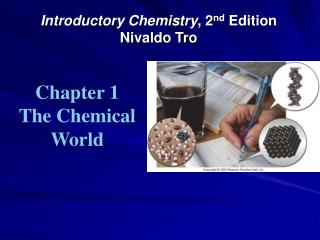DownloadDownload PresentationIntroductory Chemistry , 2 nd Edition Nivaldo Tro

# Introductory Chemistry , 2 nd Edition Nivaldo Tro

Télécharger la présentation## Introductory Chemistry , 2 nd Edition Nivaldo Tro

- - - - - - - - - - - - - - - - - - - - - - - - - - - E N D - - - - - - - - - - - - - - - - - - - - - - - - - - -
##### Presentation Transcript

1. Introductory Chemistry, 2nd EditionNivaldo Tro Chapter 1 The Chemical World

2. Why Does Soda Pop Fizz? • Everything around you is composed of chemicals • Main chemical ingredients of soda pop: water, sugar and carbon dioxide • Properties of soda pop are due to properties of these chemicals • Sugar = Sweetness • Water = Liquid • Carbon dioxide = bubbles Tro's Introductory Chemistry, Chapter 1

3. Structure Determines Properties • Everything is made of tiny particles called atoms • Properties of a substance are determined by the kinds, numbers and relationships between atoms Tro's Introductory Chemistry, Chapter 1

4. What is Chemistry? • Chemists try to discover the relationships between the particle structure of matter and the properties of matter. • Chemistry is the science that seeks to understand what matter does by studying what atoms and molecules do. Tro's Introductory Chemistry, Chapter 1

5. The Scientific Method • A process for understanding nature by observing nature and the way it behaves, and by conducting experiments to test ideas. • Parts of the Scientific Method: • Observation • Formulation of Hypotheses, • Experimentation • Formulation of Laws and Theories Tro's Introductory Chemistry, Chapter 1

6. Observation • A way of acquiring information about nature • Some observations are simple descriptions of characteristics or behavior of matter • “The soda pop is a liquid with a brown color and a sweet taste. Bubbles are seen floating up through it.” • Some observations are quantitative • “A 240 mL serving of soda pop contains 27 g of sugar.” Tro's Introductory Chemistry, Chapter 1

7. Hypothesis • A tentative interpretation or explanation of your observations • “the sweet taste of soda pop is due to the presence of sugar” • A good hypothesis is one that can be tested to be proved wrong! • Falsifiable • One test may invalidate or support your hypothesis Tro's Introductory Chemistry, Chapter 1

8. Experiments • Tests of hypotheses, laws or theories • Can you think of a way to test whether the sweet taste of soda pop is due to the presence of sugar? • Results either Validate (confirm) or Invalidate (deny) your ideas • Invalidate = Discard or Modify • Many times experiments invalidate only parts of the hypothesis or theory, in which case the idea is modified • Validate ≠ Proof your idea will always hold Tro's Introductory Chemistry, Chapter 1

9. Law • A statement summarizing all past observations: Law of Conservation of Mass – “In a chemical reaction matter is neither created nor destroyed.” • Allows prediction of future observations • So you can test the Law with experiments • Unlike state laws, you cannot choose to violate a scientific law! Tro's Introductory Chemistry, Chapter 1

10. Theory • Explanation for the characteristics and behavior of nature supported by a lot of experimental evidence • Models of nature • Dalton’s Atomic Theory • Can be used to predict future observations • So they can be tested by experiments Tro's Introductory Chemistry, Chapter 1

11. What’s the Difference Between a Hypothesis and a Theory? • A hypothesis is an explanation of a single or small number of observations • A theory is an explanation supported by a large body of experimental evidence validated by numerous scientists Tro's Introductory Chemistry, Chapter 1

12. What’s the Difference Between aLaw and a Theory? • Laws answer the question: What will happen? A summary statement; does not explain. • Theories answer the question: Why does something happen? Provide explanations. • Which will allow you to predict what will happen! Tro's Introductory Chemistry, Chapter 1

13. The Scientific Method Flow Chart Tro's Introductory Chemistry, Chapter 1

14. 2. Hypothesis 1. Observation try to further validate 3. Experiment a) conclusion try to disprove b) submission c) peer review d) publication e) other scientists respond 4. Scientific theory How Scientific Method is Followed 1. Observation 3. Experiment a) conclusion b) submission c) peer review d) publication e) other scientists respond

15. How to Succeed in Chemistry • Be Curious and use your Imagination • explore and investigate • Quantify and Calculate • even small differences can be important! • Commitment • Work Regularly & Carefully Tro's Introductory Chemistry, Chapter 1

16. Guide to Learning Chemistry • Learn the vocabulary of chemistry • Definitions of terms • How common vocabulary is applied to chemistry • Memorize important information • Names, formulas and charges of polyatomic ions • Learn and practice processes • Systematic names and formulas • Dimensional analysis (conversions) • Do the questions and exercises in the chapter to test your understanding and help you learn the patterns Tro's Introductory Chemistry, Chapter 1